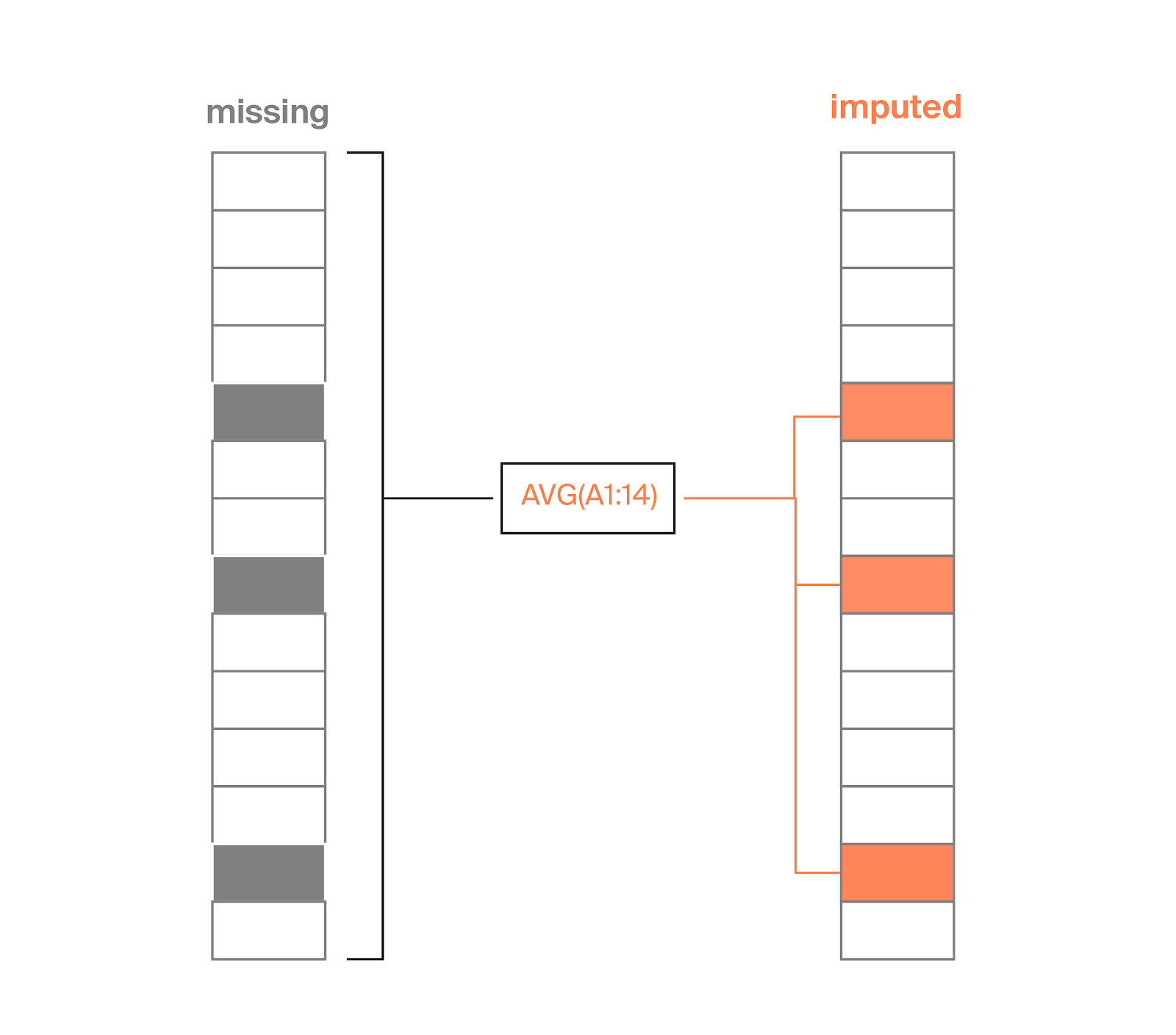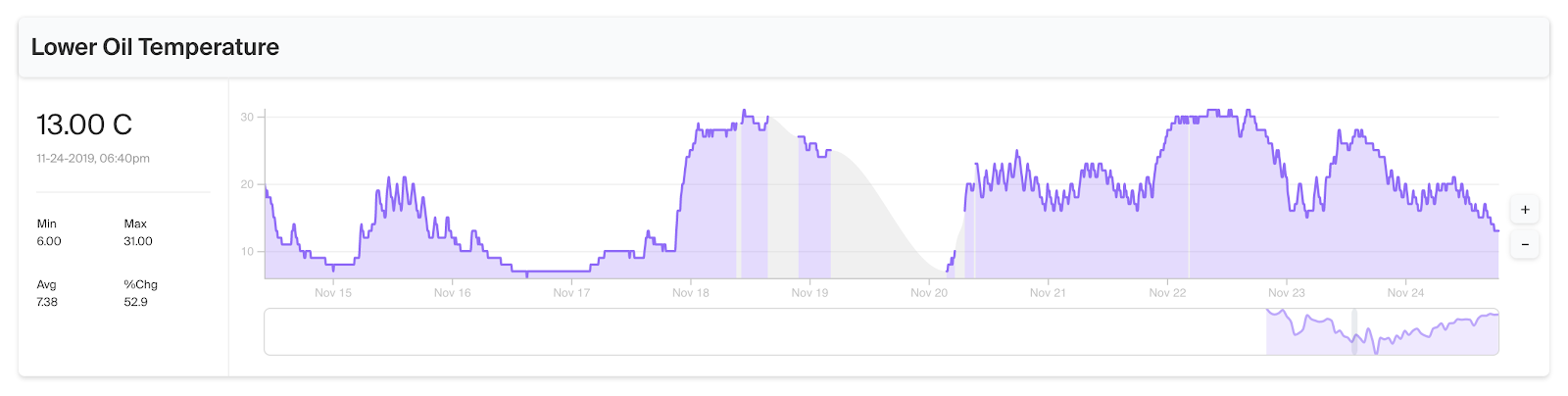# An Introduction to Imputation: Solving problems of missing and insufficient data

Missing data is a common problem. Here’s how imputation can help.

For many data scientists, analysts, or engineers, dealing with missing or bad data is an everyday experience. A dataset could have missing values for a key period of time, or perhaps the dataset contains outlier values that need to be corrected.

Often, you may look for new data or work with small subsets of the dataset. However, the complete data set, after correcting for its limitations, can hold real insights. What can you do to preserve the integrity of the data while still mining it for useful signal?

Imputation can help solve this problem. Over the coming weeks, the Tagup team will publish several articles in a series on imputation, its applications, and how to apply it on a practical level.

In this article, we’ll briefly discuss what imputation is, how it can be useful, and how imputation using machine learning models differs from other standard methods of dealing with missing or insufficient data.

# The process of imputation

Imputation can be thought of as the process of looking at a row of missing data and then “inferring”, or making a reasonable guess, as to what value should be in its place. In fact, you may have been doing imputation for a long time without knowing the name. You can replace missing data in many ways such as taking a running average or using interpolation between values. A common and simple form of model-based imputation is called “mean imputation”: when you see a missing value in a dataset, you simply take the average value for the entire column of data and insert it for all missing data points.Diagram showing the process of applying mean imputation to a column of data.

All methods of imputation have different sets of pros and cons (discussed later in the article). Basically, you can think of imputation as a set of rules: if a dataset contains missing values, apply a certain calculation to create a “best guess” replacement.

# Why imputation is useful

Data that is ideal for imputation comes in many different forms — NaN values, infrequent timestamp records, and improperly formatted numbers, to name a few. But even with these flaws, there still could be significant insight in the existing dataset. With imputation, new signals can be found in datasets with missing data (among other data quality limitations).

Imputation is a tool to recoup and preserve valuable data.

Imputation allows you to:

• Troubleshoot what may be happening in periods of missing data by simulating possible values
• Synchronize time scales for machine learning/modeling
• Smooth extremely noisy dataA sample measurement from the Tagup application, showing a period of missing data at the gray shaded regions of the chart. This data should be considered pre-imputation; for raw data in this chart, we are only applying interpolation (for predicting values at unsampled locations) between two data points instead of model-based imputation (to fill in missing values with new values).

For example, imputation can be used to fill in missing sensor measurements if you lose data communication for a day. By identifying the time range (one day) and frequency of expected measurements, you can use imputation to simulate what “normal” operating conditions would look like for this time.

# Imputation with machine learning

There are a variety of imputation methods to consider. Machine learning provides more advanced methods of dealing with missing and insufficient data compared with traditional methods. We will be covering some of these advantages in detail throughout our upcoming series on data imputation. A few existing methods include:

• Mean or median imputation
• Imputation using most frequent values
• Linear regression imputation
• Multivariate imputation by chained equation (MICE)
• k-nearest neighbors algorithm (k-NN)
• Hidden Markov Model (HMM)

For now, it’s useful to consider the following example: say you are monitoring a fleet of assets for a critical threshold alarm and you lose data communications for one of many sensor measurements. The missing data totals to about 5% of the total time range. A traditional method of imputation, such as using the mean or perhaps the most frequent value, would fill in this 5% of missing data based on the values of the other 95%.

But this traditional approach has an inherent risk: alarms and thresholds are infrequent and often short. Certain “spikes” or “anomalies” in data, by their very nature, cannot be predicted based on what is considered an average value in the dataset.

Machine learning methods such as the k-nearest neighbors algorithm (k-NN) or Hidden Markov Model (HMM) provide a more complex set of calculations for imputation. Unlike traditional methods, it also gives you more imputing abilities such as:

• Accounting for correlation between different features, rather than treating them separately
• Accounting for uncertainty bounds
• Imputing categorical values as well as numerical

In future posts within this series, we’ll break down in more detail the various applications of imputation using machine learning. We will also look at how to best visualize imputation results, and how to create and tune an imputation model.Published
July 29, 2020